## Concave Mirrors

Concave Mirrors

We give the definition of concave mirrors in previous sections. Now we will examine the reflection of light from this type of mirrors and image formation in concave mirrors. Let’s start with the reflection of light with special examples.

1. In this example, ray coming parallel to the principal axis reflects from the mirror and passes from the focal point. As in the case of plane mirrors light obey the law of reflection. In this case normal of the mirror is the line coming from the center of the mirror. If you want to draw the reflection of any light you can use this technique. Draw your normal and with the same angle of incident ray reflect your light.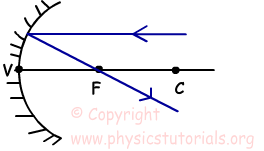2. Ray coming from the focal point reflects from the mirror and goes parallel to the principal axis, as in the first situation.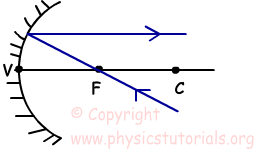3. Ray coming from the center turns back on itself after reflecting from the mirror. As I said before line coming from the center of the mirror is the normal of that system and hits the mirror perpendicularly.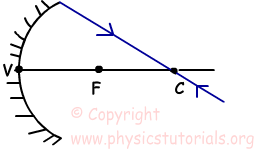4. Ray hitting the mirror at vertex point reflects with the same angle it comes.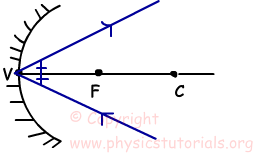5. If the ray is not one of the special rays given above, then you should draw the normal of the system and reflect the light with the same angle as it hits the mirror.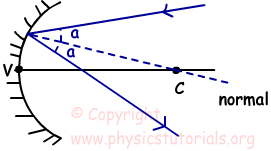Example: Find the relation between the focal lengths of the mirrors given below.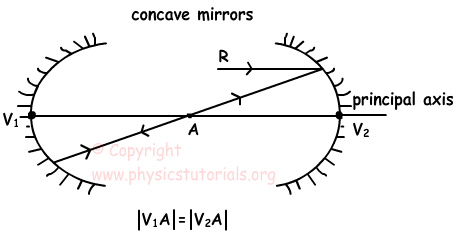Light ray R comes parallel to the principal axis and reflects from the mirror 2 and passes from the point A. We know that rays coming parallel to the principal axis passes from the focal point after reflection. Thus, point A is the focal point of the mirror 2.

Ray coming from point A turns back on itself after reflecting from mirror 1, we know that rays coming from the center of curvature turns back on itself. So, point A is the center of curvature of the mirror1.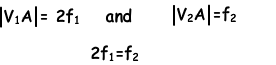Example: Look at the given system, there is a concave mirror and plane mirror located on the focal point of the concave mirror. Draw the path of the ray and show how it leaves the system.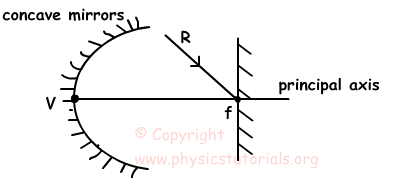Ray coming to the plane mirror reflects with the same angle, reflected ray from the mirror comes to the concave mirror from its focal point and after reflecting from the concave mirror it travels parallel to the principal axis. It comes to the plane mirror with an angle 90º, it turns back on itself and follows the same path and leaves the system.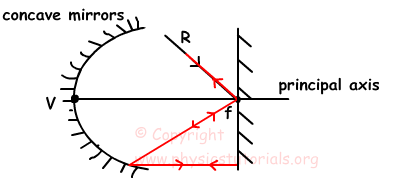Optics Exams and Solutions

Author: